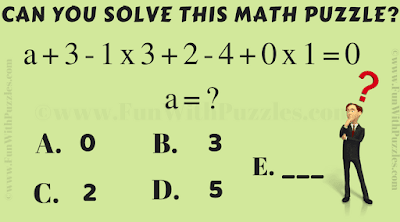This is Speed Test Brain Teaser in Mathematics in which you have to do quick Maths Calculations. Here in the given Puzzle Image, you are shown a Mathematical Equation. Your challenge is to solve this equation for a. Can you solve this Maths Speed Test Brain Teaser and find value of a?Can you solve this Speed Test Brain Teaser?

Answer of this "Maths Calculation Speed Test Brain Teaser", can be viewed by clicking on answer button. Please do give your best try before looking at the answer.

Pr@Gun said...